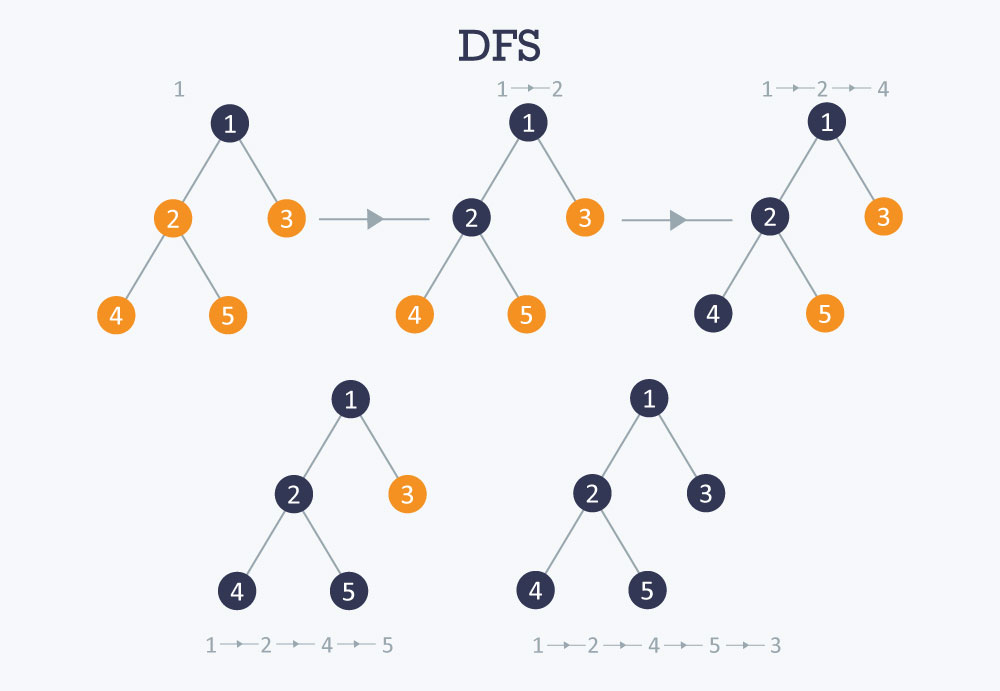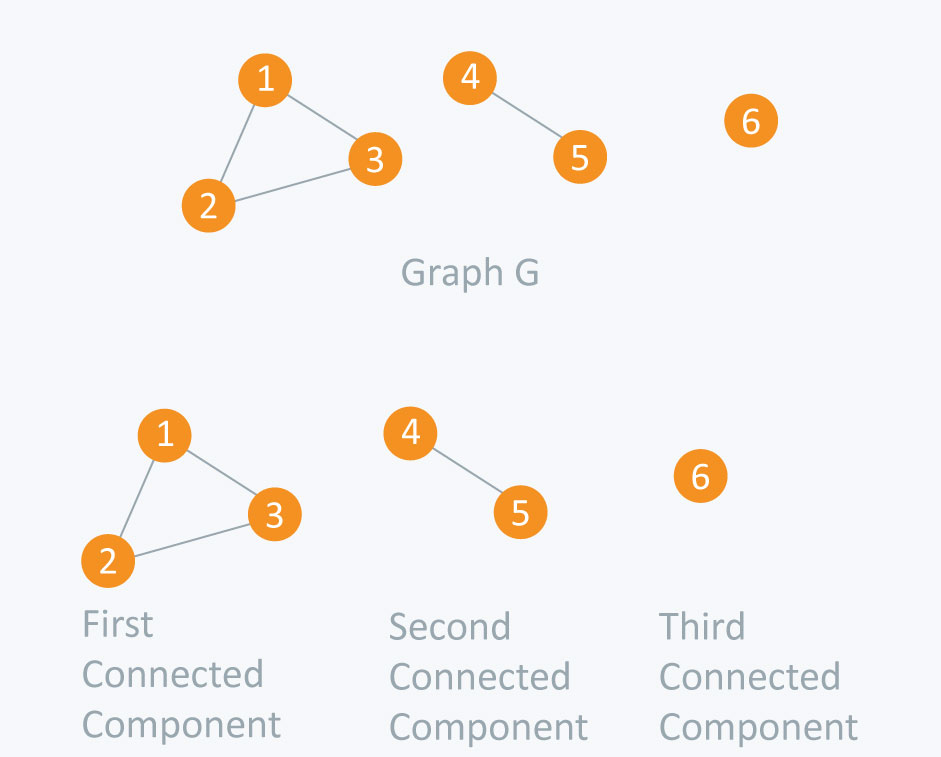Algorithms
Topics:
Depth First Search
• Searching
• Sorting
• Greedy Algorithms
• Graphs
• String Algorithms
• Dynamic Programming

# Depth First Search

Depth First Search (DFS)

The DFS algorithm is a recursive algorithm that uses the idea of backtracking. It involves exhaustive searches of all the nodes by going ahead, if possible, else by backtracking.

Here, the word backtrack means that when you are moving forward and there are no more nodes along the current path, you move backwards on the same path to find nodes to traverse. All the nodes will be visited on the current path till all the unvisited nodes have been traversed after which the next path will be selected.

This recursive nature of DFS can be implemented using stacks. The basic idea is as follows:
Pick a starting node and push all its adjacent nodes into a stack.
Pop a node from stack to select the next node to visit and push all its adjacent nodes into a stack.
Repeat this process until the stack is empty. However, ensure that the nodes that are visited are marked. This will prevent you from visiting the same node more than once. If you do not mark the nodes that are visited and you visit the same node more than once, you may end up in an infinite loop.

Pseudocode

    DFS-iterative (G, s):                                   //Where G is graph and s is source vertex
let S be stack
S.push( s )            //Inserting s in stack
mark s as visited.
while ( S is not empty):
//Pop a vertex from stack to visit next
v  =  S.top( )
S.pop( )
//Push all the neighbours of v in stack that are not visited
for all neighbours w of v in Graph G:
if w is not visited :
S.push( w )
mark w as visited

DFS-recursive(G, s):
mark s as visited
for all neighbours w of s in Graph G:
if w is not visited:
DFS-recursive(G, w)

The following image shows how DFS works.Time complexity $O (V+E)$, when implemented using an adjacency list.

Applications

How to find connected components using DFS?

A graph is said to be disconnected if it is not connected, i.e. if two nodes exist in the graph such that there is no edge in between those nodes. In an undirected graph, a connected component is a set of vertices in a graph that are linked to each other by paths.

Consider the example given in the diagram. Graph G is a disconnected graph and has the following 3 connected components.

• First connected component is 1 -> 2 -> 3 as they are linked to each other
• Second connected component 4 -> 5
• Third connected component is vertex 6

In DFS, if we start from a start node it will mark all the nodes connected to the start node as visited. Therefore, if we choose any node in a connected component and run DFS on that node it will mark the whole connected component as visited.Input File
6
4
1 2
2 3
1 3
4 5

Code

   #include <iostream>
#include <vector>
using namespace std;

bool visited;

void dfs(int s) {
visited[s] = true;
for(int i = 0;i < adj[s].size();++i)    {
}
}

void initialize() {
for(int i = 0;i < 10;++i)
visited[i] = false;
}

int main() {
int nodes, edges, x, y, connectedComponents = 0;
cin >> nodes;                       //Number of nodes
cin >> edges;                       //Number of edges
for(int i = 0;i < edges;++i) {
cin >> x >> y;
//Undirected Graph
adj[x].push_back(y);                   //Edge from vertex x to vertex y
adj[y].push_back(x);                   //Edge from vertex y to vertex x
}

initialize();                           //Initialize all nodes as not visited

for(int i = 1;i <= nodes;++i) {
if(visited[i] == false)     {
dfs(i);
connectedComponents++;
}
}
cout << "Number of connected components: " << connectedComponents << endl;
return 0;
}


Output

Number of connected components: 3

Time complexity $O (V+E)$, when implemented using the adjacency list.

Contributed by: Prateek Garg
Уведомления

?